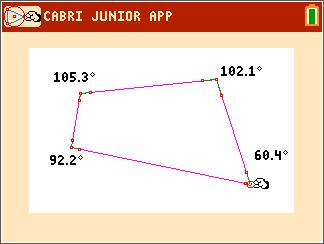### Measuring Angles in a Quadrilateral

Students determine the sum of the interior angles of a quadrilateral.
•TI-84 Plus CE
•TI-84 Plus C Silver Edition
• TI-84 Plus Silver Edition
• TI-84 Plus### Properties of Parallelograms - 1

Students explore the various properties of parallelograms and the necessary and sufficient conditions that guarantee that a quadrilateral is a parallelogram.
•TI-84 Plus CE
•TI-84 Plus C Silver Edition
• TI-84 Plus Silver Edition
• TI-84 Plus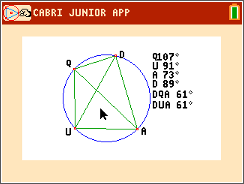Students explore various properties of cyclic quadrilaterals.
•TI-84 Plus CE
•TI-84 Plus C Silver Edition
• TI-84 Plus Silver Edition
• TI-84 Plus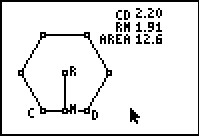### From the Center of the Polygon

Students explore the area of a regular polygon in terms of the apothem and the perimeter in order to derive the formula for a regular pentagon and regular hexagon.
•TI-84 Plus CE
•TI-84 Plus C Silver Edition
• TI-84 Plus Silver Edition
• TI-84 Plus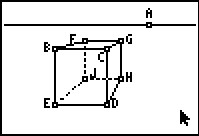### Perspective

Students draw figures in one and two-point perspective and compare and contrast the two types of drawings. They then create an isometric drawing and compare it to their drawings in perspective.
•TI-84 Plus CE
•TI-84 Plus C Silver Edition
• TI-84 Plus Silver Edition
• TI-84 Plus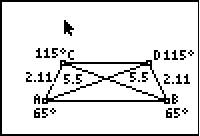### Properties of Trapezoids and Isosceles Trapezoids

A trapezoid is a quadrilateral where one pair of sides is parallel while the other two sides are not. In an isosceles trapezoid the non-parallel sides are congruent. In this activity we will attempt to create an isosceles trapezoid from an ordinary trapezoid, then approach the problem in a different manner and finally, examine the properties of trapezoids.
•TI-84 Plus CE
•TI-84 Plus C Silver Edition
• TI-84 Plus Silver Edition
• TI-84 Plus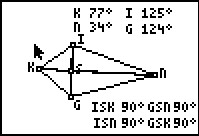••